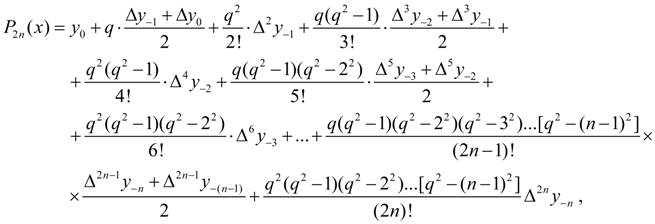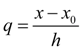Contents >> Applied Mathematics >> Numerical Methods >> Interpolation of Functions >> Stirling’s interpolation formula

 Interpolation of functions - Stirling’s interpolation formula Stirling’s interpolation formula Stirling’s interpolation formula looks like:(5) where, as before,. There are also Gauss's, Bessel's, Lagrange's and others interpolation formulas. Formula (5) is deduced with use of Gauss’s first and second interpolation formulas .

 < Previous Contents Next >